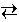# Chemical Equilibria

## Le Châtelier's Principle

### Concepts

The first step in the conversion of coal into gasoline is the steam reforming reaction.

C (s) + H2O (g)CO (g) + H2 (g)

Suppose that 0.0500 mole each of carbon, water, carbon monoxide, and hydrogen are placed in a 10.0 L glass bulb, which is then heated to 1000 K and the steam reforming reaction is allowed to reach equilibrium. This system is depicted in the experiment shown below.

The question to be answered in this experiment is:

How does a change in the analytical amount of a reactant or product affect the equilibrium amounts of reactants and products in the system?

The distinction between analytical amount and equilibrium amount is especially important in this experiment. The analytical amount of material, such as carbon, is the amount physically added to the system. The equilibrium amount is the amount of material that actually exists in the system at equilibrium. The two amounts are generally not the same, because the reaction will consume or produce the material in order to reach equilibrium.

In this experiment, for example, the analytical amount of each reactant and product is 0.0500 mole, but the equilibrium amounts of carbon and water are 0.0093 mole and the equilibrium amounts of carbon monoxide and hydrogen are 0.0907 mole. One consequence of the dynamic nature of the system is that the amount of a compound placed in a system is rarely the amount that actually exists in the system.

### Experiment

Objective:

Explore the effect of changing the analytical amounts of reactants and products on the equilibrium composition of the system.

Part 1 Experiment

The reset button resets the analytical amounts of each material to 0.0500 mole. For each material a slider is provided that permits the analytical amount of that material to be dynamically changed. The system automatically reacts to this change and reaches a new equilibrium state. The equilibrium amounts of each material are shown in the graph and in the boxes along the bottom of the display.

Perform the following experiments and carefully observe the behavior of the system.

1. Reset the experiment. Use the bottom slider to increase the analytical amount of hydrogen and observe how the equilibrium amounts of each species change. Obviously the equilibrium amount of hydrogen increase. What happens to the equilibrium amounts of carbon, water, and carbon monoxide? Decrease the amount of hydrogen and observe the results.

2. Reset the experiment. Use the second slider to decrease the analytical amount of water and observe how the equilibrium amounts of each species change.

3. Reset the experiment. Use the equilibrium amounts, the system volume of 10.0 L, and the system temperature of 1000. K to calculate the equilibrium constant, KP, for the reaction. Vary the amounts of carbon monoxide and hydrogen and repeat the calculation. You should always obtain the same value.

 Analytical Amounts of Material placed in the Glass Bulb moles C moles H2O moles CO moles H2 Equilibrium Amounts of Material in the Glass Bulb C moles           H2O moles           CO moles           H2 moles

Part 1. Discussion

Did you find a pattern in the behavior of the system in Part 1 of the experiment?

The French chemist Henri-Louis Le Châtelier summarized this behavior in what is now called Le Châtelier's Principle:

When a stress is brought to bear on a system at equilibrium, the system will react in the direction that serves to relieve the stress.

Basically, whenever you make a change to a system at equilibrium, nature tries to undo the change.

Suppose the system is at equilibrium with 0.0500 mole analytical amounts of each reactant and product. When additional hydrogen is added to the system, this constitutes a stress on the system that pushes the system away from equilibrium. To return to equilibrium, nature attempts to reduce the amount of hydrogen (that is, it tries to undo the addition of hydrogen) by having the hydrogen react with carbon monoxide to produce carbon and water. Thus when hydrogen is added to the system, the equilibrium amount of carbon monoxide decreases and the equilibrium amounts of carbon and water increase.

The converse behavior occurs when the amount of a reactant is changed. If necessary, repeat the experiment and look for this behavior.

Part 2. Experiment and Discussion

Depending upon the conditions you explored, you may have found unusual behavior associated with carbon. To see how carbon is unusual, perform the following experiments with the system shown above.

1. Reset the experiment. Use the top slider to increase the analytical amount of carbon in the system. What happens to the equilibrium amounts of water, carbon monoxide, and hydrogen?

Why are the equilibrium amounts of water, carbon monoxide, and hydrogen unaffected by the analytical amount of carbon? (See below for the exception.)

2. Reset the experiment. Use the top slider to slowly decrease the analytical amount of carbon in the system. Initially the equilibrium amounts of the other species do not change. At what analytical amount of carbon do the equilibrium amounts of the other compounds in the reaction begin to decrease? Why does this behavior occur?

3. Reset the experiment. Use the top slider to set the analytical amount of carbon to 0.0200 mole. What is the equilibrium amount of carbon? Is it appropriate to talk about the steam reforming reaction being at equilibrium under this condition? Use the equilibrium amounts to calculate the equilibrium constant. Do you obtain the same value as in Part 1?Reaction Table                     Le Châtelier's Principle: Changes in VolumeChemical Equilibria Home PageVirtual Chemistry Home Page

LeChatelier.html version 2.11